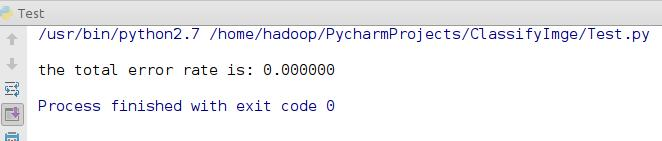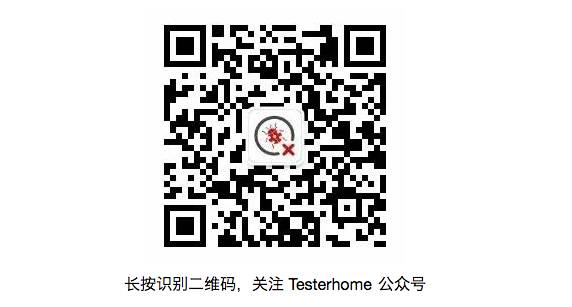工具选择

• Python机器学习包，Scikit-learn，超好用的机器学习包，一直都在用这个机器学习包。

工欲善其事必先利其器

• 楼主的Python版本是2.7，已经安装好pip，所以只要简单几步就可以安装好运行Scikit-learn所需要的环境

• 安装Numpy
\$ sudo pip install numpy
• 安装Scipy
\$ sudo pip install scipy
• 安装Scikit-learn
\$ sudo pip install scikit-learn
• 安装PIL， 这个用来读取图片，到官网下载安装包到本地，切换到解压路径
\$ sudo python setup.py install

注意： 有可能还要安装JPEG的包，这个取决于本机是否已经安装，未安装的可以参考网上教程

其他

• 训练数据以及测试数据，这里楼主自拍了7种不同插件的照片共计136张，测试数据5种共计5张

对于机器学习来说，数据是越多越好越多越好，更多的数据会让你的模型效果更佳 ：）

• 你仅仅需要一点点机器学习的基础知识，以及Python基础知识。

那我们就从这里开始进入正文

1. KNN

使用KNN的时候，因为数据样本不够多，正确率大概在60%左右，不是太可观的结果。

2. SVM
对于，支持向量机，也使用了两种算法

1. SVC："one-against-one"策略
2. LinearSVC："one-vs-the-rest"策略

import Image
import numpy as np
import os
from sklearn import svm

def getTrainClass(category):
if category == 'Train':
classes = {0, 1, 2, 3, 4, 5, 6, 7}
else:
classes = {0, 1, 2, 5, 7}
return classes

def getImageData(path,classes):
ImageList = []
LabelList = []
for name in classes:
class_path = cwd + path + "/" + str(name) + "/"
for image_name in os.listdir(class_path):
image_path = class_path + image_name
if 'JPG' in image_path:
img = Image.open(image_path)
ImageList.append(np.asarray(img).flatten())
LabelList.append(name)
return ImageList, LabelList

TrClasses = getTrainClass('Train')
tr_img, tr_label = getImageData('Train',TrClasses) #加载训练数据
tstClass = getTrainClass('Validation')
tst_img, tst_label = getImageData('Validation',tstClass) #加载测试数据

svcClf = svm.LinearSVC() #直接使用默认参数就好了
svcClf.fit(tr_img,tr_label)

Z = svcClf.predict(tst_img)

result = (1 - np.sum(Z==tst_label)/float(len(tst_img)))
print "\nthe total error rate is: %f" %result写在最后的话↙↙↙阅读原文可查看相关链接，并与作者交流